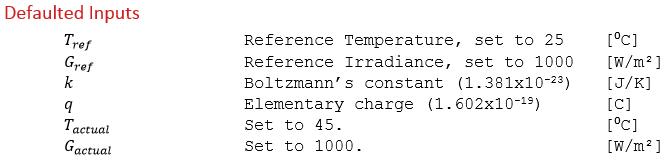# Linear Temperature Dependence of Diode Ideality Factor Calculation

This algorithm calculates the linear temperature dependence of the diode ideality factor. In general, it does this by determining a value that results in a temperature coefficient of maximum power as close as possible to the datasheet input.1. Determine the direction that mu gamma should increment.

The linear temperature dependence of gamma is incremented once in both the positive and negative directions, creating a set of three values:For each of these values, γ is temperature adjusted to 45⁰C, in addition to temperature adjusting all of the other single diode parameter inputs (see the algorithm documentation section entitled “One-Diode Model Temperature Correction”).

Calculate Pmp at STC then for each of the three mu gamma values, calculate Pmp at 45⁰C and calculate mu_Pmp using the following equation:If the error between calculated mu_Pmp and mu_Pmp from the datasheet input decreases for mu_gamma + increment, then mu_gamma should be incremented in the positive direction to achieve the optimal value.

1. Iterative increment to the optimal value that generates close to the inputted value of mu_Pmp.

Increment mu_gamma in the direction determined in step 1, performing a similar calculation to step 1 and checking the error between calculated_mu_Pmp and mu_Pmp at each iteration. The optimal value of mu_Pmp that which precedes the error between calculated_mu_Pmp and mu_Pmp starting to increase.